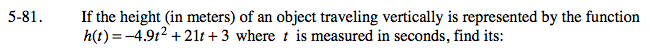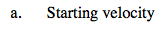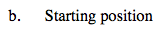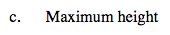### Home > CALC > Chapter 5 > Lesson 5.2.3 > Problem5-81

5-81.Evaluate v(t) when t = 0.Evaluate h(t) when t = 0.Since the height function is a parabola, the maximum height can be determined by using Algebra to locate the vertex. Calculus can also be used.

The maximum height will occur at the time when v(t) = 0.

The vertex of the parabola is located at t = –21/2(–4.9) ≈ 2.143 seconds.
h(2.143) ≈ 25.5 m